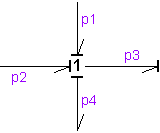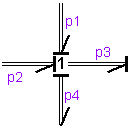﻿ 20-sim webhelp > Library > Bond Graph > onejunction

# onejunction

Bond Graph

## Use

Domains: Continuous. Size: [n,m]. Kind: Bond Graphs.

## Description

This junction represents a power continuous (no energy storage, dissipation or generation) connection of elements. The sum of the efforts on all ports is zero and the flows on all ports are equal. The constitutive equations are for example:p1.e + p2.e - p3.e - p4.e = 0;   p1.f = p2.f = p3.f = p4.f;   flow = p4.f;

A one junction has only one initial port p defined. Because any number of bonds may be connected, successive connected bonds are named p1, p2, p3 etc.

The plus or minus signs of the effort equation depend on the direction of the bonds. A bond pointing towards the one junction results in a plus sign and vice-versa. Only one port of a one junction may determine the flow. In the example port p4 determines the flow.

## Multi Bonds

Bonds with a size larger than one (multi bonds) can also be connected to a one junction. Al connected bonds, however, must have the same size.p1.e + p2.e - p3.e + p4.e = 0;   p1.f = p2.f = p3.f = p4.f;   flow = p4.f;

Naming conventions are equal to those of the single bond (size 1) junctions. Successive connected bonds are named p1, p2, p3 etc. To denote single elements of a multibond, matrix notation is used. E.g. the effort of bond number 3 of a multibond p2 can be denoted as p2.e (columnvector notation) or p2.e[3,1] (matrix notation).

## Interface

Ports

Description

p[any]

Any number of ports may be connected.

## Causality

1_effort p

Only one port may have an effort out causality (as seen from the element).

## Outputs

flow

The output signal is equal to the flow of the ports.

## Limitations

All connected bonds must have the same size.